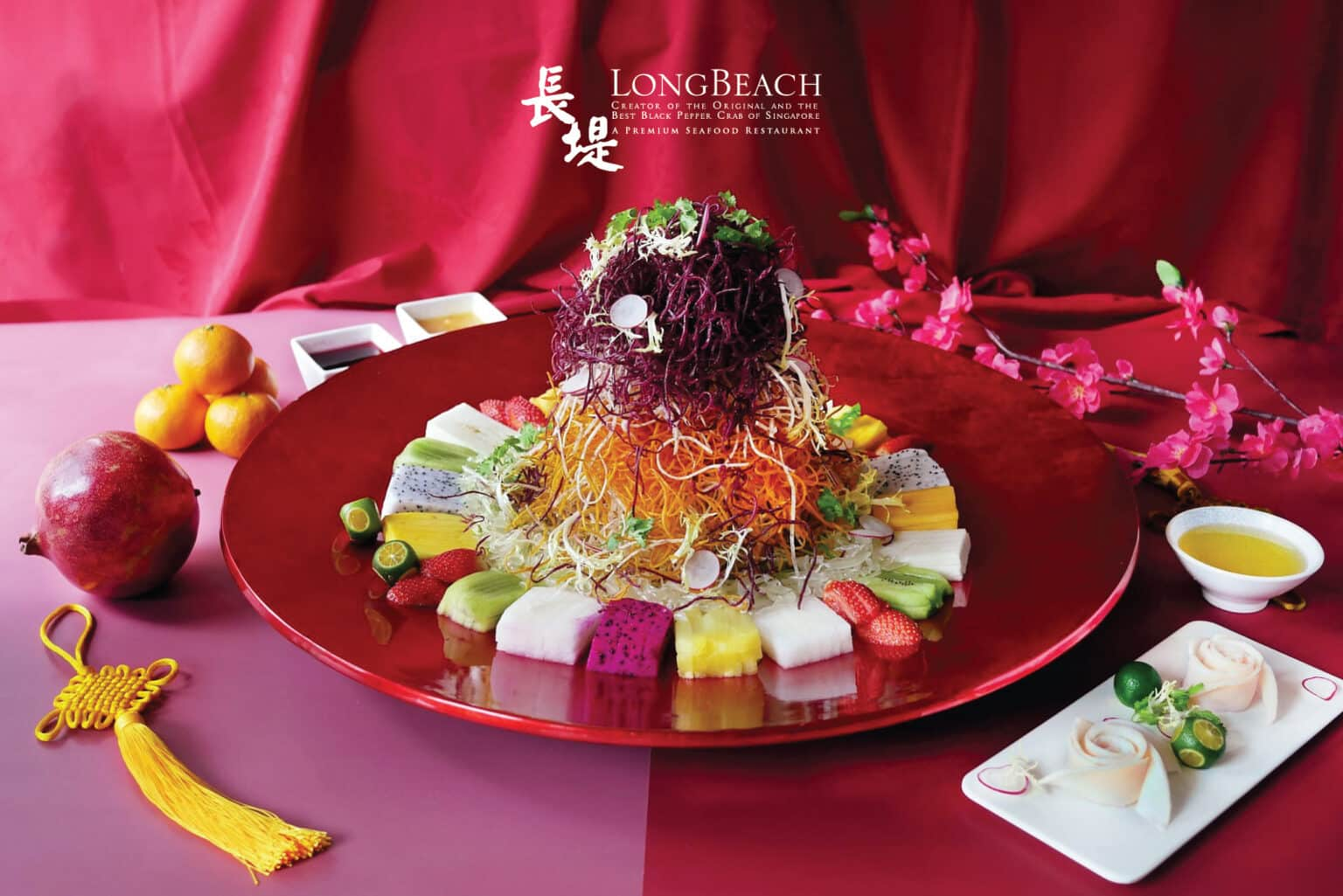Days
Hours
Minutes
Seconds
Happy Year of the Rabbit!

## YEAR OF THE RABBIT @ LONG BEACH

Reserve a table now
Long Beach wishes all a Prosperous Chinese New Year

Let’s ring in the Year of the Rabbit with a Chinese New Year Reunion Dinner | 团圆饭 at Long Beach.

*CNY Opening hours | Weekday, 23(Mon) & 24(Tues) January | All outlets will be open From 11am – 11pm

## 👇 Check out our CNY menu 👇

DempseyBringing back an all-time favourite, the Fruits Yusheng is Long Beach’s healthier alternative to the festive platter. To ensure the quality and the freshness of the seasonal fruits, our chefs will decide which seasonal fruits such as Dragon Fruit, Jackfruit, Mango and more, to be served on that day.

🦀Fruits Yusheng is available at \$XXX++ (serves eight) and above | Limited availability | For dine-in only. An advance order of three days is required.

## Experience Chinese New Year with Long Beach

### How to get to . ?

X X X X X X X X X X X X X X X X X X X X X X X X X X X X X X X X X X X X X X X X X X X X X X X X X X X X X X X X X X X X X X X X X X X X X X X X X X X X X X X X X X X  X X X X X X X X X X X X X X X X X X X X X X X X X X X X X X X X X X X X X X X X X X X X X X X X X X X X X X X X X X X X X X X X X X X X X X X X X X X X X X X X X X X